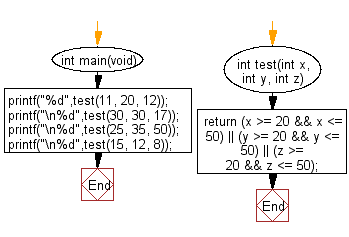﻿ C Program: Test three integer values are within 20..50# C Exercises: Check whether three given integer values are in the range 20..50 inclusive

## C-programming basic algorithm: Exercise-8 with Solution

Write a C program to check whether three given integer values are in the range 20..50 inclusive. Return true if 1 or more of them are in the said range otherwise return false.

C Code:

``````#include <stdio.h>
#include <stdlib.h>
int main(void){
printf("%d",test(11, 20, 12));
printf("\n%d",test(30, 30, 17));
printf("\n%d",test(25, 35, 50));
printf("\n%d",test(15, 12, 8));
}
int test(int x, int y, int z)
{
return (x >= 20 && x <= 50) || (y >= 20 && y <= 50) || (z >= 20 && z <= 50);
}
``````

Sample Output:

```1
1
1
0
```

Pictorial Presentation:Flowchart:C Programming Code Editor:

What is the difficulty level of this exercise?

Test your Programming skills with w3resource's quiz.

﻿

## C Programming: Tips of the Day

Why doesn't a+++++b work?

printf("%d",a+++++b); is interpreted as (a++)++ + b according to the Maximal Munch Rule!.

++ (postfix) doesn't evaluate to an lvalue but it requires its operand to be an lvalue.

! 6.4/4 says the next preprocessing token is the longest sequence of characters that could constitute a preprocessing token"

Ref : https://bit.ly/3fdldUT